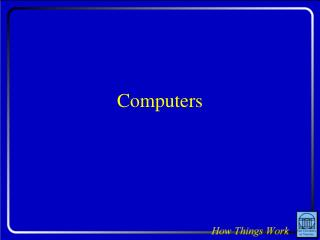# Computers - PowerPoint PPT PresentationDownload PresentationComputers

ComputersDownload Presentation## Computers

- - - - - - - - - - - - - - - - - - - - - - - - - - - E N D - - - - - - - - - - - - - - - - - - - - - - - - - - -
##### Presentation Transcript

1. Computers

2. Question: Today, the fastest PCs run at roughly 1.5 GHz. Someday, computers may run at 1,000,000 GHz. Compared to present computers, those high-speed ones would have to be • much larger. • much smaller. • about the same size.

3. Observations About Computers • They respond to inputs with various outputs • They handle all kinds of information • Information is measured in bits and bytes • Some information is lost when power fails • Computers work extremely quickly • They follow instructions perfectly

4. Analog Representation • A number is represented by a physical quantity • Current • Voltage • Magnetization • Number is proportional to the physical quantity • Precision is determined by the quantity itself

5. Digital Representation • A numbers is represent by physical quantities • Physical quantities take on discrete values • These values represent pieces of the number • Precision is determined by number of quantities

6. Binary Representation • Each physical quantity has two values • One value is defined as a “1” • The other value is defined as a “0” • Each quantity represents one information bit • A number is represented by several bits • The more bits, the more precision • Bits are relatively immune to noise

7. Example: 19 • Five bits can represent number from 0 to 31 • 19 is represented by the bits: 10011 • Each bit represents a power of 2 • 1·24 + 0·23 + 0·22 + 1·21 + 1·20 = 19

8. Representing Non-Numbers • Bits or groups of bits are assigned to objects • Characters • Colors • Days of the week • 8 bits (a byte) can distinguish 256 objects • Two bytes can distinguish 65,536 objects

9. Quantities Representing Bits • Current • Magnetization • Charge • Optical properties • Light • Radio Waves • Sound

10. Computers & Bits • Computation: currents • Memory: charge • Disk Drives: magnetization • CDROM/DVDROM: optical properties

11. Computing • Computers perform logical operations with bits • Complicated operations based on simple ones • Simplest operations: inversion & not-and • Any function can be realized from these two

12. Inverter • Takes one input bit, provides one output bit • Output bit is inverse of input bit

13. Not-And (NAND) • Takes two input bits, provides one output bit • Output is inverse of logical “and” of input bits

14. CMOS Logic • Bits are represented by charge • “1” is represented by positive charge • “0” is represented by negative or no charge • Logic is built from n-channel and p-channel MOSFETS in complementary pairs

15. CMOS Inverter • Input charge delivered to two complementary MOSFETs • Positive charge on input delivers negative charge to output • Vice Versa

16. CMOS NAND • Positive on both inputs delivers negative charge to output • Negative on either input delivers positive charge to output

17. Personal Computers • Use CMOS logic for computations • Use charge-based memory for fast storage • Use magnetization or optical for slow storage • Use light, radio, current, or sound for network

18. Speed Limits • Bits move no faster than the speed of light • Speed of light is 1 foot per nanosecond • During one PC cycle, bits can move 1 foot • Processors can’t be bigger than 1 foot

19. Question: Today, the fastest PCs run at roughly 1.5 GHz. Someday, computers may run at 1,000,000 GHz. Compared to present computers, those high-speed ones would have to be • much larger. • much smaller. • about the same size.